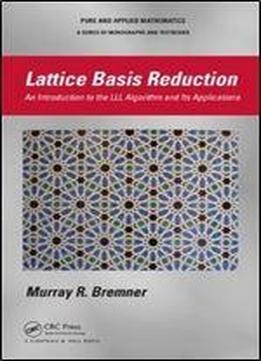# Lattice Basis Reduction: An Introduction To The Lll Algorithm And Its Applications by Murray R. Bremner / 2011 / English / PDF

First developed in the early 1980s by Lenstra, Lenstra, and Lovsz, the LLL algorithm was originally used to provide a polynomial-time algorithm for factoring polynomials with rational coefficients. It very quickly became an essential tool in integer linear programming problems and was later adapted for use in cryptanalysis. This book provides an introduction to the theory and applications of lattice basis reduction and the LLL algorithm. With numerous examples and suggested exercises, the text discusses various applications of lattice basis reduction to cryptography, number theory, polynomial factorization, and matrix canonical forms.

views: 589